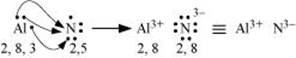### Chemical Bonding and Molecular Structure - Solutions

CBSE Class 11 Chemistry

NCERT Solutions
Chapter 4
CHEMICAL BONDING AND MOLECULAR STRUCTURE

1. Explain the formation of a chemical bond.

Ans. A chemical bond is defined as an attractive force that holds the constituents (atoms, ions etc.) together in a chemical species.

Various theories have been suggested for the formation of chemical bonds such as the electronic theory, valence shell electron pair repulsion theory, valence bond theory, and molecular orbital theory.

A chemical bond formation is attributed to the tendency of a system to attain stability. It was observed that the inertness of noble gases was because of their fully filled outermost orbitals. Hence, it was postulated that the elements having incomplete outermost shells are unstable (reactive). Atoms, therefore, combine with each other and complete their respective octets or duplets to attain the stable configuration of the nearest noble gases. This combination can occur either by sharing of electrons or by transferring one or more electrons from one atom to another. The chemical bond formed as a result of sharing of electrons between atoms is called a covalent bond. An ionic bond is formed as a result of the transference of electrons from one atom to another.

2. Write Lewis dot symbols for atoms of the following elements: Mg, Na, B, O, N, Br.

Ans. Mg: There are two valence electrons in Mg atom. Hence, the Lewis dot symbol for Mg is: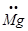Na: There is only one valence electron in an atom of sodium. Hence, the Lewis dot structure is: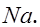B: There are 3 valence electrons in Boron atom. Hence, the Lewis dot structure is: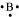O: There are six valence electrons in an atom of oxygen. Hence, the Lewis dot structure is: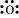N: There are five valence electrons in an atom of nitrogen. Hence, the Lewis dot structure is: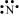Br: There are seven valence electrons in bromine. Hence, the Lewis dot structure is: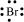3. Write Lewis symbols for the following atoms and ions:

(i) S and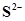;

(ii) Al and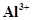;

(iii) H and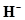Ans. (i) S and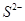The number of valence electrons in sulphur is 6.

The Lewis dot symbol of sulphur (S) is

The dinegative charge infers that there will be two electrons more in addition to the six valence electrons. Hence, the Lewis dot symbol ofis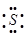.

(ii) Al and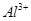The number of valence electrons in aluminium is 3.

The Lewis dot symbol of aluminium (Al) is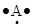.

The tripositive charge on a species infers that it has donated its three electrons. Hence, the Lewis dot symbol is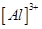.

(iii) H and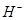The number of valence electrons in hydrogen is 1.

The Lewis dot symbol of hydrogen (H) is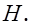The uninegative charge infers that there will be one electron more in addition to the one valence electron. Hence, the Lewis dot symbol is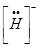.

4. Draw the Lewis structures for the following molecules and ions: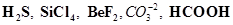Ans.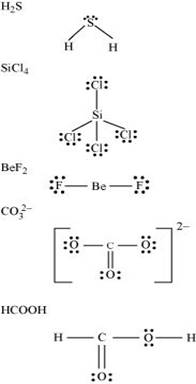5. Define octet rule. Write its significance and limitations.

Ans. The octet rule or the electronic theory of chemical bonding was developed by Kossel and Lewis. According to this rule, atoms can combine either by transfer of valence electrons from one atom to another or by sharing their valence electrons in order to attain the nearest noble gas configuration by having an octet in their valence shell.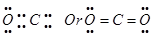The octet rule successfully explained the formation of chemical bonds depending upon the nature of the element.

Limitations of the octet theory:

The following are the limitations of the octet rule:

(a) The rule failed to predict the shape and relative stability of molecules.

(b) It is based upon the inert nature of noble gases. However, some noble gases like xenon and krypton form compounds such as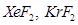etc.

(c) The octet rule cannot be applied to the elements in and beyond the third period of the periodic table. The elements present in these periods have more than eight valence electrons around the central atom. For example: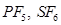, etc.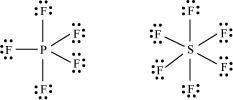(d) The octet rule is not satisfied for all atoms in a molecule having an odd number of electrons. For example, NO and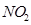do not satisfy the octet rule.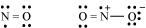(e) This rule cannot be applied to those compounds in which the number of electrons surrounding the central atom is less than eight. For example, LiCl,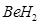,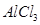etc. do not obey the octet rule.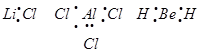6. Write the favourable factors for the formation of ionic bond.
Ans. An ionic bond is formed by the transfer of one or more electrons from one atom to another. Hence, the formation of ionic bonds depends upon the ease with which neutral atoms can lose or gain electrons. Bond formation also depends upon the lattice energy of the compound formed.

Hence, favourable factors for ionic bond formation are as follows:

(i) Low ionization enthalpy of metal atom.

(ii) High electron gain enthalpy (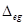H) of a non-metal atom.

(iii) High lattice energy of the compound formed.

7. Discuss the shape of the following molecules using the VSEPR model: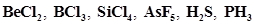Ans.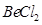: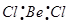The central atom has no lone pair and there are two bond pairs. i.e., BeCl2 is of the type AB2. Hence, it has a linear shape.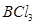: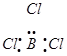The central atom has no lone pair and there are three bond pairs. Hence, it is of the type AB3. Hence, it is trigonal planar.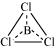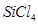: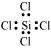The central atom has no lone pair and there are four bond pairs. Hence, the shape of SiCl4 is tetrahedral being the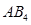type molecule.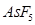: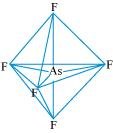The central atom has no lone pair and there are five bond pairs. Hence, AsF5 is of the type AB5. Therefore, the shape is trigonal bipyramidal.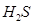: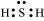The central atom has one lone pair and there are two bond pairs. Hence, H2S is of the type AB2E. The shape is Bent.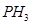: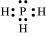The central atom has one lone pair and there are three bond pairs. Hence, PH3 is of the AB3E type. Therefore, the shape is trigonal pyramidal.

8. Although geometries of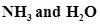molecules are distorted tetrahedral, bond angle in water is less than that of ammonia. Discuss.

Ans. The molecular geometry ofcan be shown as: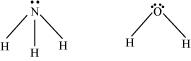The central atom (N) in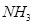has one lone pair and there are three bond pairs. In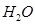, there are two lone pairs and two bond pairs.

The two lone pairs present in the oxygen atom ofmolecule repels the two bond pairs. This repulsion is stronger than the repulsion between the lone pair and the three bond pairs on the nitrogen atom.

Since the repulsion on the bond pairs inmolecule are greater than that in, the bond angle in water is less than that of ammonia.

9. How do you express the bond strength in terms of bond order?

Ans. Bond strength represents the extent of bonding between two atoms forming a molecule. The larger the bond energy, the stronger is the bond and the greater is the bond order.

10. Define the bond length.

Ans. Bond length is defined as the equilibrium distance between the nuclei of two bonded atoms in a molecule.

Bond lengths are expressed in terms of Angstrom (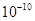m) or picometer

(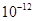m) and are measured by spectroscopic X-ray diffraction and electron-diffraction techniques.

In an ionic compound, the bond length is the sum of the ionic radii of the constituting atoms (d =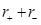). In a covalent compound, it is the sum of their covalent radii (d =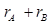).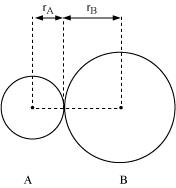11. Explain the important aspects of resonance with reference to the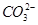ion.

Ans. According to experimental findings, all carbon to oxygen bonds inare equivalent. Hence, it is inadequate to represention by a single Lewis structure having two single bonds and one double bond.

Therefore, carbonate ion is described as a resonance hybrid of the following structures: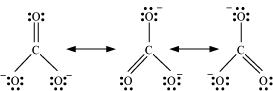12.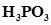can be represented by structures 1 and 2 shown below. Can these two structures be taken as the canonical forms of the resonance hybrid representing? If not, give reasons for the same.

Ans. The given structures cannot be taken as the canonical forms of the resonance hybrid ofbecause the positions of the atoms have changed.

13. Write the resonance structures for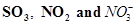.

Ans. The resonance structures are:

(a)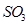:(b)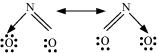(c)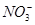: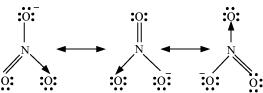14. Use Lewis symbols to show electron transfer between the following atoms to form cations and anions: (a) K and S (b) Ca and O (c) Al and N.

Ans. (a) K and S:

The electronic configurations of K and S are as follows:

K: 2, 8, 8, 1

S: 2, 8, 6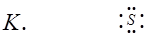Sulphur (S) requires 2 more electrons to complete its octet. Potassium (K) requires one electron more than the nearest noble gas i.e., Argon. Hence, the electron transfer can be shown as: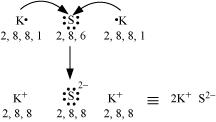(b) Ca and O:

The electronic configurations of Ca and O are as follows:

Ca: 2, 8, 8, 2

O: 2, 6

Oxygen requires two electrons more to complete its octet, whereas calcium has two electrons more than the nearest noble gas i.e., Argon. Hence, the electron transfer takes place as: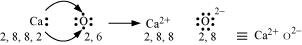(c) Al and N:

The electronic configurations of Al and N are as follows:

Al: 2, 8, 3

N: 2, 5

Nitrogen is three electrons short of the nearest noble gas (Neon), whereas aluminium has three electrons more than Neon. Hence, the electron transference can be shown as: